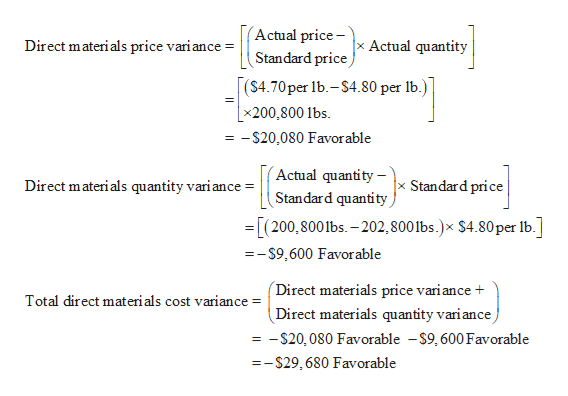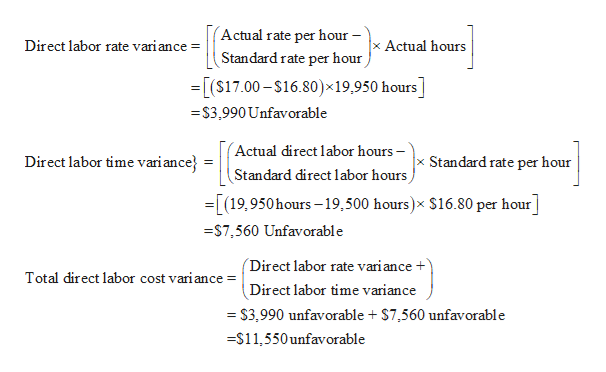Question
200 views

Direct Materials, Direct Labor, and Reports budgeted and actual costs for variable and fixed factory overhead along with the related controllable and volume variances.Factory Overhead Cost Variance Analysis

Mackinaw Inc. processes a base chemical into plastic. A detailed estimate of what a product should cost.Standard costs and actual costs for direct materials, direct labor, and factory overhead incurred for the manufacture of 78,000 units of product were as follows:

 Standard Costs Actual Costs Direct materials 202,800 lbs. at \$4.80 200,800 lbs. at \$4.70 Direct labor 19,500 hrs. at \$16.80 19,950 hrs. at \$17.00 Factory overhead Rates per direct labor hr., based on 100% of normal capacity of 20,350 direct labor hrs.: Variable cost, \$4.40 \$84,940 variable cost Fixed cost, \$7.00 \$142,450 fixed cost

Each unit requires 0.25 hour of direct labor.

Required:

a.  Determine the direct materials Price variance is the difference between the actual and standard prices, multiplied by the actual quantity.price variance, direct materials The cost associated with the difference between the standard quantity and the actual quantity of direct materials used in producing a commodity.quantity variance, and total direct materials The difference between actual cost and standard cost at actual volumes.cost variance. Enter a favorable variance as a negative number using a minus sign and an unfavorable variance as a positive number.

 Direct Materials Price Variance \$ Favorable    Favorable Unfavorable Direct Materials Quantity Variance \$ Favorable    Favorable Unfavorable Total Direct Materials Cost Variance \$ Favorable    Favorable Unfavorable

b.  Determine the direct labor The cost associated with the difference between the standard rate and the actual rate paid for direct labor used in producing a commodity.rate variance, direct labor The cost associated with the difference between standard and actual hours of direct labor spent for producing a commodity.time variance, and total direct labor cost variance. Enter a favorable variance as a negative number using a minus sign and an unfavorable variance as a positive number.

 Direct Labor Rate Variance \$ Unfavorable    Favorable Unfavorable Direct Labor Time Variance \$ Unfavorable    Favorable Unfavorable Total Direct Labor Cost Variance \$ Unfavorable    Favorable Unfavorable

c.  Determine the variable factory overhead The difference between the actual variable overhead costs and the budgeted variable overhead for actual production.controllable variance, fixed factory overhead The difference between the budgeted fixed overhead at 100% of normal capacity and the standard fixed overhead for the actual production achieved during the period.volume variance, and total factory overhead cost variance. Enter a favorable variance as a negative number using a minus sign and an unfavorable variance as a positive number.

 Variable factory overhead controllable variance \$ Favorable    Favorable Unfavorable Fixed factory overhead volume variance \$ Unfavorable    Favorable Unfavorable Total factory overhead cost variance \$ Unfavorable    Favorable Unfavorable

check_circle

Step 1

a.help_outlineImage TranscriptioncloseActual price- xActual quantity Direct materials price vari ance = Standard price (S4.70per lb.-\$4.80 per 1b.) |x200,800 lbs = -S20,080 Favorable Actual quantity - Standard price Direct materials quantity variance Standard quantity [(200,8001bs. -202, 800 Ibs.)x \$4.80per Ib. --S9,600 Favorable Direct materials price variance Total direct materials cost variance = Direct materials quantity variance = -S20,080 Favorable -S9,600Favorable =-S29 ,680 Favorable fullscreen
Step 2

b.

...help_outlineImage TranscriptioncloseActual rate per hour - Direct labor rate variance Actual hours Standard rate per hour [(S17.00-\$16.80)x 19,950 hours] S3,990 Unfavorable Actual direct labor hours -' Standard direct labor hours Direct labor time variance x Standard rate per hour (19,950hours-19,500 hours)x \$16.80 per hour - S7 ,560 Unfavorable (Direct labor rate vari ance Total direct labor cost variance = Direct labor time variance =S3,990 unfavorable \$7,560 unfavorable -S11,550unfavorable fullscreen

### Want to see the full answer?

See Solution

#### Want to see this answer and more?

Solutions are written by subject experts who are available 24/7. Questions are typically answered within 1 hour.*

See Solution
*Response times may vary by subject and question.
Tagged in